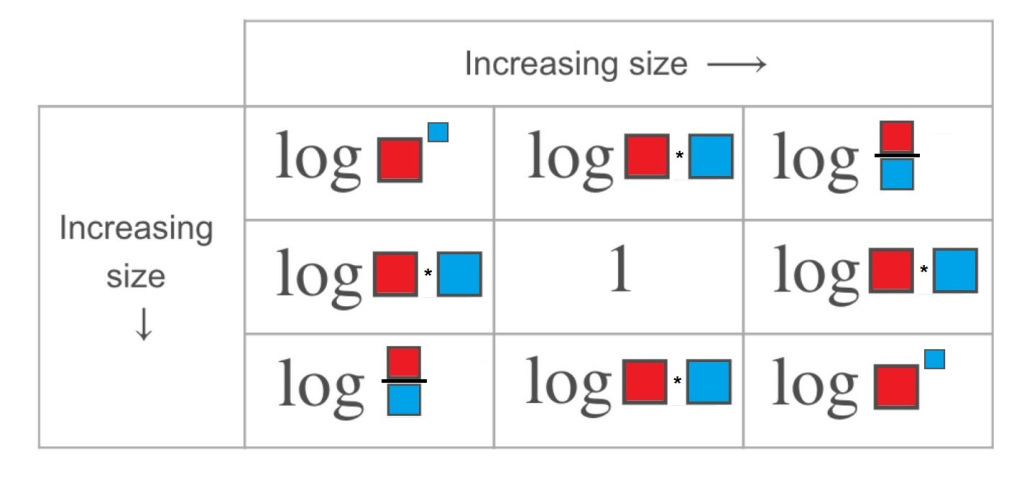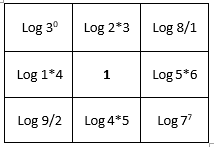Home > High School: Functions > Laws of Logarithms

# Laws of Logarithms

Directions: Using the integers 0 to 9, fill in the red and blue boxes so that the chart is accurate. You can only use a number once per red box and once per blue box. (Logs are in base 10)### Hint

What mathematical equation can be produced from a logarithm? How does that relate to the size of a logarithm?
How can you rewrite the log of a product, quotient, or power?

There are many possible solutions. One example would be:One thing to watch is the use of 0, many students will not recognize that Log 0 is undefined

Source: Bryan Anderson

## Creating Sequences

Directions: Using the digits 0-9, at most one time each, complete the first three terms …

1.2.